# MetaTrader 4 indicator – Hourly Pivot Points with Color

5/4/2018 update:

• Fixed an error in calculating S/R points by Fibo.
• Fixed a bug in drawing objects.

This indicator uses two ways to calculate R1/R2/R3/S1/S2/S3: normal formula and Fibonacci, and users can choose one from them.

### Main parameters

• PivotMode:
• Normal: use normal formula to calculate S and R.
• Fibonacci: use Fibonacci to calculate S and R.

.

• CurrentHourOnly: only calculate lastet hourly PP if true.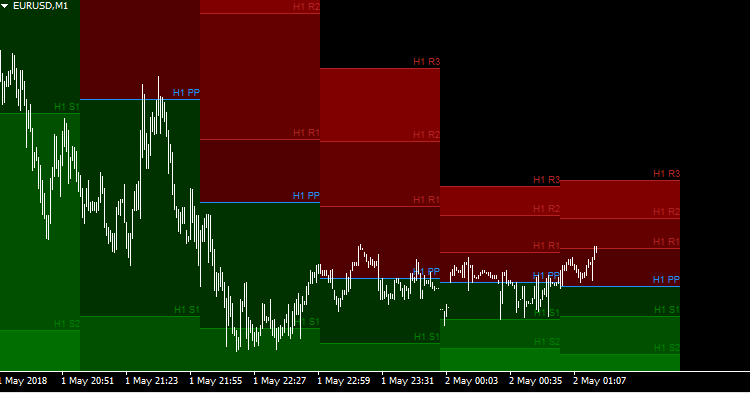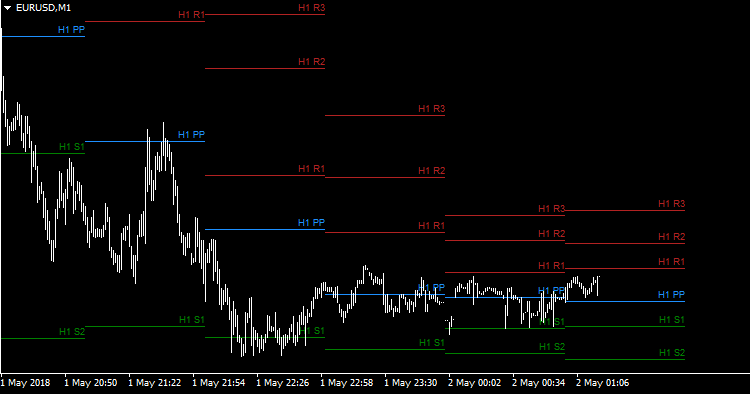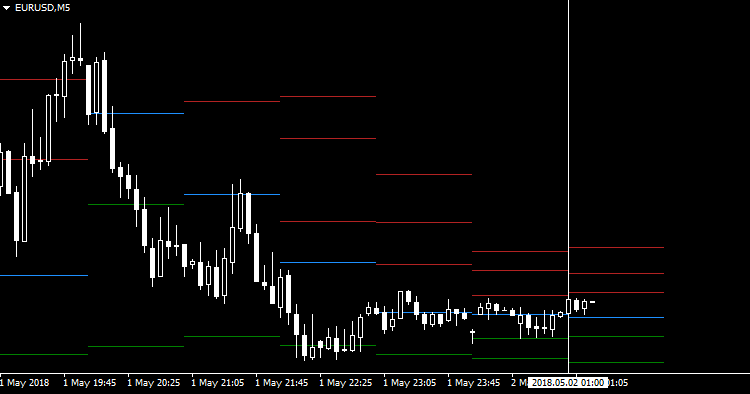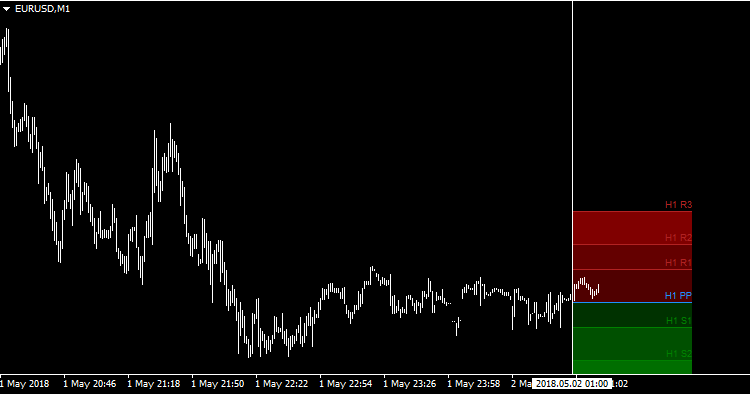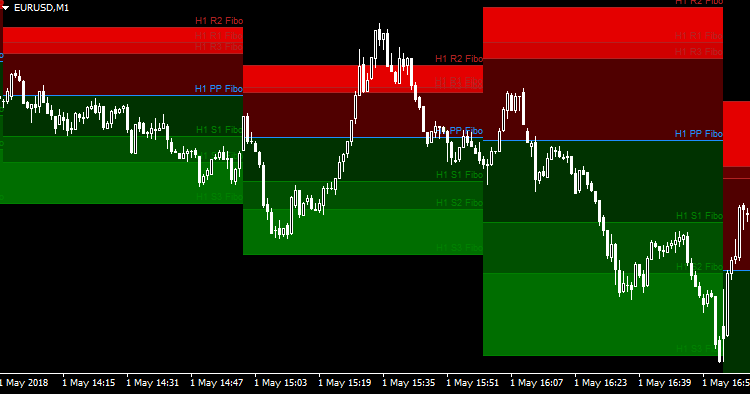[/viral_lock_button]